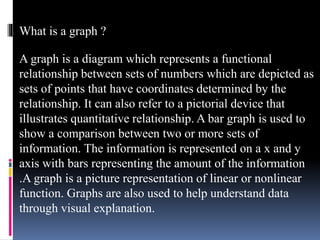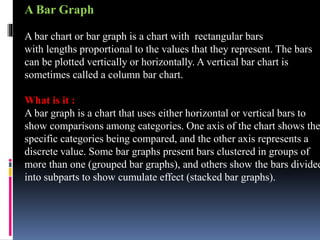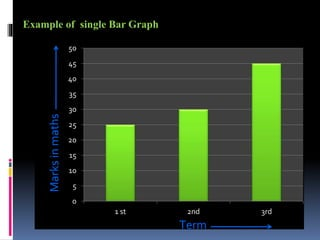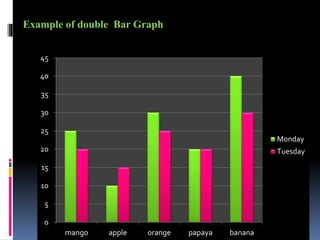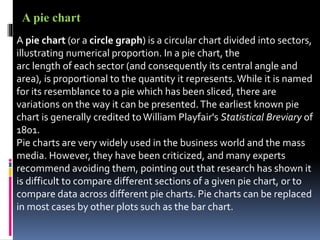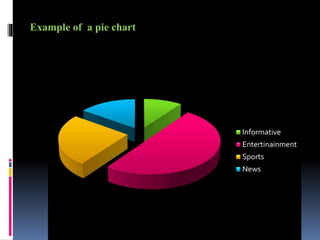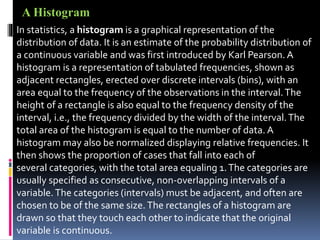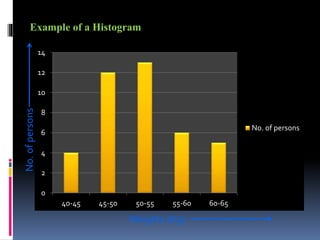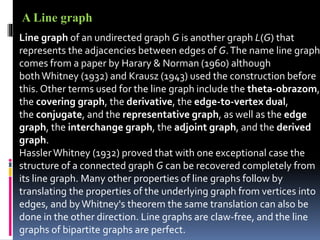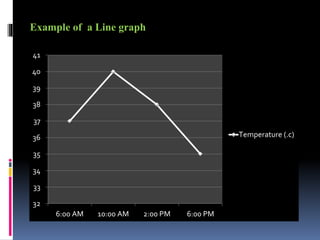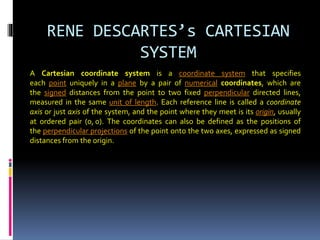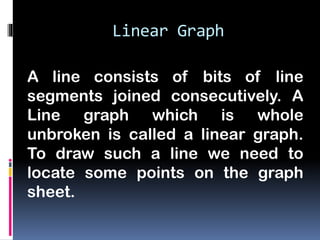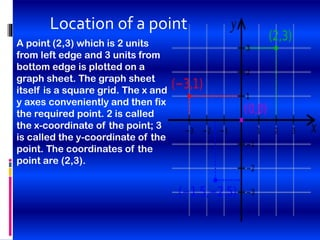1 de 22

### Introduction to graph

1. Introduction To Graph
2. What is a graph ? A graph is a diagram which represents a functional relationship between sets of numbers which are depicted as sets of points that have coordinates determined by the relationship. It can also refer to a pictorial device that illustrates quantitative relationship. A bar graph is used to show a comparison between two or more sets of information. The information is represented on a x and y axis with bars representing the amount of the information .A graph is a picture representation of linear or nonlinear function. Graphs are also used to help understand data through visual explanation.
3. A Bar Graph A bar chart or bar graph is a chart with rectangular bars with lengths proportional to the values that they represent. The bars can be plotted vertically or horizontally. A vertical bar chart is sometimes called a column bar chart. What is it : A bar graph is a chart that uses either horizontal or vertical bars to show comparisons among categories. One axis of the chart shows the specific categories being compared, and the other axis represents a discrete value. Some bar graphs present bars clustered in groups of more than one (grouped bar graphs), and others show the bars divided into subparts to show cumulate effect (stacked bar graphs).
4. 0 5 10 15 20 25 30 35 40 45 50 1 st 2nd 3rd Marksinmaths Term Example of single Bar Graph
5. Example of double Bar Graph 0 5 10 15 20 25 30 35 40 45 mango apple orange papaya banana Monday Tuesday
6. A pie chart (or a circle graph) is a circular chart divided into sectors, illustrating numerical proportion. In a pie chart, the arc length of each sector (and consequently its central angle and area), is proportional to the quantity it represents. While it is named for its resemblance to a pie which has been sliced, there are variations on the way it can be presented.The earliest known pie chart is generally credited toWilliam Playfair's Statistical Breviary of 1801. Pie charts are very widely used in the business world and the mass media. However, they have been criticized, and many experts recommend avoiding them, pointing out that research has shown it is difficult to compare different sections of a given pie chart, or to compare data across different pie charts. Pie charts can be replaced in most cases by other plots such as the bar chart. A pie chart
7. Example of a pie chart Informative Entertinainment Sports News
8. In statistics, a histogram is a graphical representation of the distribution of data. It is an estimate of the probability distribution of a continuous variable and was first introduced by Karl Pearson. A histogram is a representation of tabulated frequencies, shown as adjacent rectangles, erected over discrete intervals (bins), with an area equal to the frequency of the observations in the interval.The height of a rectangle is also equal to the frequency density of the interval, i.e., the frequency divided by the width of the interval.The total area of the histogram is equal to the number of data. A histogram may also be normalized displaying relative frequencies. It then shows the proportion of cases that fall into each of several categories, with the total area equaling 1.The categories are usually specified as consecutive, non-overlapping intervals of a variable.The categories (intervals) must be adjacent, and often are chosen to be of the same size. The rectangles of a histogram are drawn so that they touch each other to indicate that the original variable is continuous. A Histogram
9. Example of a Histogram 0 2 4 6 8 10 12 14 40-45 45-50 50-55 55-60 60-65 No. of persons Weights (Kg) No.ofpersons
10. Line graph of an undirected graph G is another graph L(G) that represents the adjacencies between edges of G.The name line graph comes from a paper by Harary & Norman (1960) although bothWhitney (1932) and Krausz (1943) used the construction before this. Other terms used for the line graph include the theta-obrazom, the covering graph, the derivative, the edge-to-vertex dual, the conjugate, and the representative graph, as well as the edge graph, the interchange graph, the adjoint graph, and the derived graph. HasslerWhitney (1932) proved that with one exceptional case the structure of a connected graph G can be recovered completely from its line graph. Many other properties of line graphs follow by translating the properties of the underlying graph from vertices into edges, and byWhitney's theorem the same translation can also be done in the other direction. Line graphs are claw-free, and the line graphs of bipartite graphs are perfect. A Line graph
11. Example of a Line graph 32 33 34 35 36 37 38 39 40 41 6:00 AM 10:00 AM 2:00 PM 6:00 PM Temperature (.c)
12. RENE DESCARTES
13. RENE DESCARTES’s CARTESIAN SYSTEM A Cartesian coordinate system is a coordinate system that specifies each point uniquely in a plane by a pair of numerical coordinates, which are the signed distances from the point to two fixed perpendicular directed lines, measured in the same unit of length. Each reference line is called a coordinate axis or just axis of the system, and the point where they meet is its origin, usually at ordered pair (0, 0). The coordinates can also be defined as the positions of the perpendicular projections of the point onto the two axes, expressed as signed distances from the origin.
14. Linear Graph A line consists of bits of line segments joined consecutively. A Line graph which is whole unbroken is called a linear graph. To draw such a line we need to locate some points on the graph sheet.
15. Location of a point A point (2,3) which is 2 units from left edge and 3 units from bottom edge is plotted on a graph sheet. The graph sheet itself is a square grid. The x and y axes conveniently and then fix the required point. 2 is called the x-coordinate of the point; 3 is called the y-coordinate of the point. The coordinates of the point are (2,3).
16. I HOPEYOU HAVE UNDERSTOOD WHAT I HAVETAUGHT. THANKYOU FOR COORDINATING.
17. THANK YOU
18. GROUP MEMBERS I. Aditya D. Patil II. Bikrant Roy III. Abhisek IV. Akash Kumar V. Akshay P.C VI. Akshay Dani